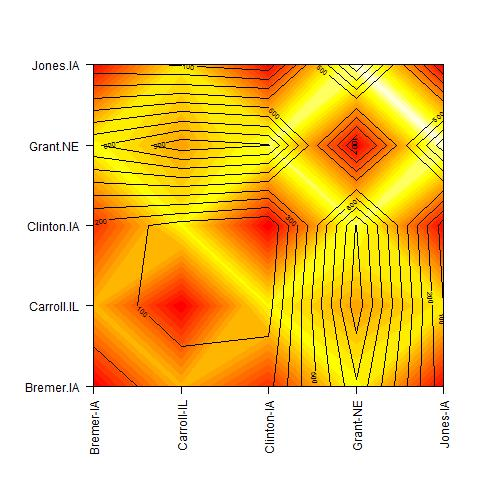# IBE/IBD Contour plots in R

Rob’s post from yesterday motivated me to find an alternate way of visualizing correlations between matrices of geographical or ecological data, and genetic data. I have seen plenty of Mantel, or partial Mantel tests of correlation, as well as plots of “IBD”, or Isolation By Distance (which plots some genetic distance versus some ecological/geographical distance), but how fun would it be if you could visualize these correlations between sampling locales in different variables (here genetic differentiation, genetic distances, geographical distances, ecological distances, etc) on the same plot? So that’s exactly what I set out to do.Contour plot of correlated geographical (black) and ecological distances (heat map), from Manthey and Moyle (2015).

As an example, I downloaded the data from Table 2 of Manthey and Moyle (2015), which shows geographical distances versus ‘ecological’ distances. As a second example, I also downloaded data from Table 2 of Sethuraman et al. (2014), which shows population pairwise differentiation (Fst), versus geographical distances. I constructed symmetric matrices from these, and used the modified “filled.contour2” function (modified version of filled.contour) for these plots. So, assuming that you have symmetric matrices saved as text files (you can download these examples from this link),

```#Function courtesy http://tinyurl.com/oqxt8dq
matrix.axes <- function(data) {
x <- (1:dim(data) - 1) / (dim(data) - 1);
axis(side=1, at=x, labels=rownames(data), las=2);
x <- (1:dim(data) - 1) / (dim(data) - 1);
axis(side=2, at=x, labels=colnames(data), las=2);
}
source(“http://tinyurl.com/psdtkgr”)
par(mar=c(6,6,4,2)+0.5)
filled.contour2(n1,plot.axes=matrix.axes(n1))
```Contour plot of geographical distance (black) versus genetic differentiation (heat map) from the data of Sethuraman et al. (2013).

And voila! I modified the code above with different color palettes for the data of Sethuraman et al. (2013). I find these plots a lot more informative than say Figure 3 of Manthey and Moyle (2015), or Figure 2 of Sethuraman et al. (2013). What are your thoughts? Do let me know! Ideally, I’d like to have this contour plot over a geographical map – but I’ll save that for a future post. Good luck!